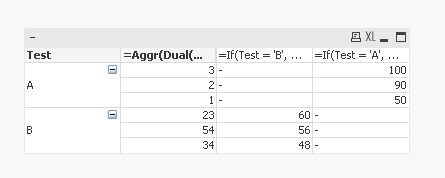QlikView App Development

Discussion Board for collaboration related to QlikView App Development.

Highlighted
New Contributor III

Need help in dual function

Hi,

I've a pivot table like below in my Qlikview app.

 Test Server sum(sales1) Sum(Sales) A 1 50 2 90 3 100 B 23 60 34 48 54 56

I would like to sort the sum(sales) based on server dimension and I'm using below expression in the sort tab of the pivot table.

dual(Test='A',sum(sales))

And I'm getting the output as below.

 Test Server sum(sales1) Sum(Sales) A 3 100 2 90 1 50 B 23 60 34 48 54 56

Now my requirement is I would like to sort both the 'Tests(A and B)' and I'm trying the below expression and is giving me the error message saying it is not possible.

dual(Test='A',sum(sales),

dual(Test='B',sum(sales1))

I would like to see output as below,

 Test Server sum(sales1) Sum(Sales) A 3 100 2 90 1 50 B 23 60 54 56 34 48

Regards,

Vikas

7 RepliesMVP

Re: Need help in dual function

May be

dual(Test='A' or Test='B',sum(sales))

Before develop something, think If placed (The Right information | To the right people | At the Right time | In the Right place | With the Right context)
New Contributor III

Re: Need help in dual function

Sorry Anil, I just edited my questionMVP

Re: Need help in dual function

Why 34 and 54 interchange?

Before develop something, think If placed (The Right information | To the right people | At the Right time | In the Right place | With the Right context)
New Contributor III

Re: Need help in dual function

I want that sorted based on the highest value, so that's why they got interchanged.MVP

Re: Need help in dual function

I see you have changed your requirement a little bit, so you don't want this any more?Dimension

Test

=Aggr(Dual(Server, Sum(Sales)), Test, Server)

Expression

=If(Test = 'B', Sum(Sales))

=If(Test = 'A', Sum(Sales))

Sort tab

Make sure to sort numerically the second dimension by descending order

New Contributor III

Re: Need help in dual function

No sunny, I'm using different expression sum(sales) and sum(sales1).MVP

Re: Need help in dual function

How does it matter....Changed the data to this

Test, Server, Sales, Sales1

A, 1, 50

A, 2, 90

A, 3, 100

B, 23, , 60

B, 34, , 48

B, 54, , 56

];

and use this

Dimension

Test

=Aggr(Dual(Server, Sum(Sales)+Sum(Sales1)), Test, Server)

Expression

=If(Test = 'B', Sum(Sales1))

=If(Test = 'A', Sum(Sales))